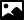## Data Category diffrn_scan_frame_axis

### GeneralCategory name
diffrn_scan_frame_axis
Required in PDB entries
no
Category group membership
diffrn_group
Used in current PDB entries
No

### Category Relationship DiagramsView full category relationship diagram including all dictionary data categories

### Category Description```    Data items in the DIFFRN_SCAN_FRAME_AXIS category describe the
settings of axes for particular frames.  Unspecified axes are
assumed to be at their zero points.  If, for any given frame,
nonzero values apply for any of the data items in this category,
those values should be given explicitly in this category and not
simply inferred from values in DIFFRN_SCAN_AXIS.```

### NeXus Mapping Details```

_diffrn_scan_frame_axis.axis_id AXISID
_diffrn_scan_frame_axis.angle ANGLE
_diffrn_scan_frame_axis.angle_increment ANGLEINCREMENT
_diffrn_scan_frame_axis.angle_rstrt_incr ANGLERSTRTINCREMENT
_diffrn_scan_frame_axis.displacement DISP
_diffrn_scan_frame_axis.displacement_increment DISPINCREMENT
_diffrn_scan_frame_axis.displacement_rstrt_incr DISPRSTRTINCREMENT
_diffrn_scan_frame_axis.reference_angle REFANGLE
_diffrn_scan_frame_axis.reference_displacement REFDISP

{ /entry:NXentry
/CBF_scan_id="SCANID"
/instrument:NXinstrument
/DETECTORNAME:NXdetector_group
/DETECTORELEMENTNAME:NXdetector
for AXISEQUIPMENT=="detector"}
{  /entry:NXentry
/sample:NXsample
for AXISEQUIPMENT=="goniometer"}
{  /entry:NXentry
for AXISEQUIPMENT=="general"}
/transformations:NXtransformations
/AXISID=[]
@diffrn_scan_frame_axis__angle_start=[ANGSTART]
@diffrn_scan_frame_axis__angle_range=[ANGRANGE]
@diffrn_scan_frame_axis__angle_increment=[ANGINC]
@diffrn_scan_frame_axis__angle_rstrt_incr=[ANGRSTRT]
@diffrn_scan_frame_axis__displacement_start=[DISPSTART]
@diffrn_scan_frame_axis__displacement_range=[DISPRANGE]
@diffrn_scan_frame_axis__displacement_increment=[DISPINC]
@diffrn_scan_frame_axis__displacement_rstrt_incr=[DISPRSTRT]
@diffrn_scan_frame_axis__reference_angle=[ANG]
@diffrn_scan_frame_axis__reference_displacement=[DISP]

note that @units="mm" or @units="deg" should also be specified.

The dimensions of the array depend on np (the number of frames = the
value of _diffrn_scan.frames)

either DISP OR ANGLE is inserted as the i-th element
counting from 1 in AXISID where i is the value of
_diffrn_scan_frame.frame_number for which the value
of _diffrn_scan_frame.frame_id agrees with the
value of _diffrn_scan_frame_axis.frame_id

The remaining tags similarly populate the attribute arrays```